# Sig Fig Calculator

Enter the number in sig fig calculator and hit the Calculate button to find the number of significant figures.

Give Us Feedback

## Sig Fig Calculator

Sig Fig calculator is an online maths tool used for counting the number of significant digits in a number. It will tell you the reason why a digit is significant or insignificant.

## How does the significant figures calculator work?

To use the sig figs calculator, follow these instructions:

• Enter the Number.
• Enter the round value. (optional)
• Click Calculate.

This sig fig rounding calculator also gives other important information about a number. This information includes;

## What are significant figures?

According to Wikipedia:

Significant figures of a number are digits in the number that are reliable and absolutely necessary to indicate the quantity of something.”

In other words, the digits of a number or value which make a difference when used in any calculation are called significant figures.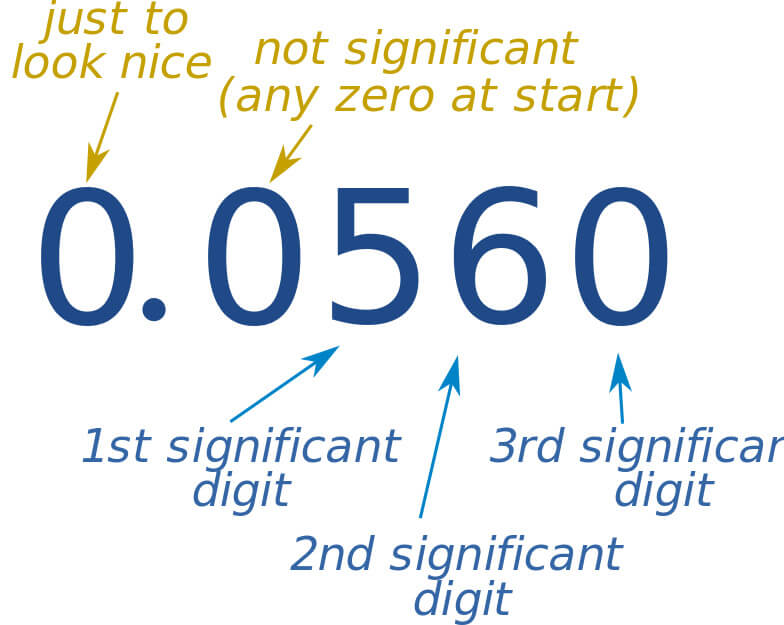## How to find significant figures?

Significant figures can be calculated using the sig figs rules.

### Rounding Significant figures rules

In this calculator, every significant figure is explained using the rule it falls on. These rules are very important. Everyone should be familiar with these rules if they want to identify significant figures on their own.

These rules are:

• ### Non- zeros

All the non-zero digits in a number, no matter at which place they are, are significant. For example: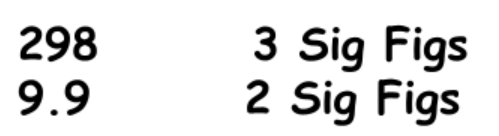• ### Zero between non-zeros

The zeros that are present between two significant figures are also significant.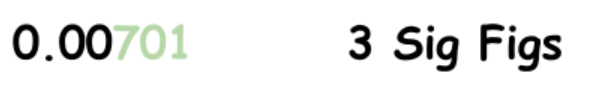The zeros that are present on the left side of a number before any significant figure are considered insignificant. In the same example as mentioned above: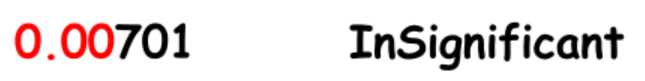• ### Trailing zeros

If the number has a decimal point then the trailing zeros are significant.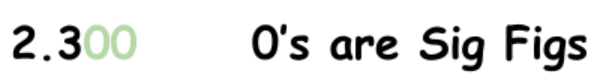But if the number is without any decimal point, trailing zeros are insignificant.## Significant figures - Examples

Identify the significant figures from these numbers.

• 12309

• 0.090
• 101.9

Solution:

`1st number: 12309`

All the digits are significant.

No. of sig figs = 5

`2nd number: 0.090`

9 and 0 after nine are significant.

No. of sig figs = 2

`3rd number: 101.9`

All the digits are significant.

No. of Sig figs = 4

### Math Tools